# Neural Correlate-Based E-Learning Validation and Classification Using Convolutional and Long Short-Term Memory Networks

Neural Correlate-Based E-Learning Validation and Classification Using Convolutional and Long Short-Term Memory Networks
Department of CSE, Amity School of Engineering and Technology, Amity University, Chhattisgarh 492001, India

Corresponding Author Email:
dharmendra.pathak@s.amity.edu
Page:
1457-1467
|
DOI:
https://doi.org/10.18280/ts.400414
16 January 2023
|
Revised:
1 April 2023
|
Accepted:
28 May 2023
|
Available online:
31 August 2023
| Citation

OPEN ACCESS

Abstract:

The COVID-19 pandemic has precipitated an unprecedented surge in the proliferation of online E-learning platforms, designed to cater to a wide array of subjects across all age groups. However, a paucity of these platforms adopts a learner-centric approach or validates user learning, underscoring the need for effective E-learning validation and personalized learning recommendations. This paper addresses these challenges by implementing an innovative approach that leverages real-time electroencephalogram (EEG) signals collected from learners, who don neuro headsets while partaking in online courses. These EEG signals are subsequently classified using Convolutional Neural Networks (CNN) and Long Short-Term Memory (LSTM) deep learning models, with the intent of discerning the efficacy of the E-learning process. The proposed models have yielded promising classification accuracies of 68% and 97% for the CNN and LSTM models, respectively, demonstrating their rapidity and precision in classifying E-learning EEG signals. Thus, these models hold substantial potential for application in similar E-learning validation scenarios. Furthermore, this study introduces an automated framework designed to track the learning curve of users and furnish valuable recommendations for E-learning materials. The presented approach, therefore, not only validates the E-learning process but also aids in optimizing the learning experiences on E-learning platforms.

Keywords:

automated framework, convolution neural network, deep learning, EEG signals, E-learning, feature extraction, Long Short-Term Memory, neuro headsets

1. Introduction

We are currently witnessing an era characterized by a burgeoning growth of technology in education. From online courses and virtual laboratories to e-tutoring, digital learning platforms have emerged as effective and economical alternatives to traditional classroom instruction, a trend catalyzed by the ongoing COVID-19 pandemic. Recent research indicates that E-learning platforms enhance student retention rates by 25% to 60% compared to conventional teaching methods, offering unparalleled flexibility in terms of time, location independence, resource availability, and ease of access .

However, despite the demonstrated efficacy of E-learning, maintaining high levels of concentration over extended periods remains a formidable challenge for users . Consequently, there is a pressing need for a framework capable of not only validating and customizing learning in real-time but also elucidating the user's learning trajectory.

Electroencephalographic (EEG) signals, the digital imprints of brain activity measured in microvolts (µV), have been proposed as a solution. These signals are characterized by specific frequencies: Delta (0Hz to 4Hz), associated with healing, deep sleep, and the immune system; Theta (4Hz to 8Hz), correlated with relaxation, creativity, and emotional states; Alpha (8Hz to 12Hz), indicative of focus and relaxation; Beta (12Hz to 40Hz), linked to problem-solving and conscious focus; and Gamma (40Hz to 100Hz), representative of acute senses, cognition, and learning. These signals can be captured using neuro headsets or consumer-grade brain-computer interface (BCI) devices, with subsequent analysis via machine learning/deep learning algorithms.

Deep learning, a subset of machine learning, employs deep neural networks to mimic the functions of the human brain for classification tasks . As depicted in Figure 1, each layer within these networks - input, hidden, and output - serves as a processing unit responsible for tasks such as feature extraction and classification. Crucial parameters governing the strength of network classification include weight, bias, and activation functions.

Deep learning's ability to handle large, complex datasets through the concept of feature hierarchy, as demonstrated in Figure 2, renders it ideal for pattern recognition, classification, and the identification of hitherto unknown patterns .

The present study explores the classification of EEG signals using Convolutional Neural Networks (CNN) and Long Short-Term Memory (LSTM) deep learning models. These models are capable of classifying real-time E-learning EEG data to validate user learning, monitor attention levels, and delineate learning patterns. Furthermore, the proposed framework offers recommendations for E-learning material customization and provides improved feedback mechanisms for individual and collaborative learning. This methodology could also serve as a potential tool for identifying learning disabilities among students.

## 1.png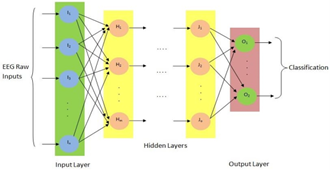Figure 1. Architecture of deep neural network

## 2.png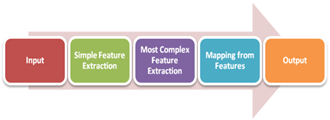Figure 2. Processing pipelines of deep neural network

2. Challenges in the Current E-Learning Scenario

The ongoing pandemic compels millions of users all over the world to shift to online educational platforms. Even with many advantages of online education in terms of time, place, and resource flexibility, there are certain critical challenges associated with e-education . Following are some major challenges associated with E-learning:

2.1 Student's low attention span

Many factors are responsible for this mainly: platform UI/UX, mode of communication, media, experts, timings, internet speed, student interest/expertise in the subjects, practical/case studies, etc. . Also, there is a requirement for an approach that can detect students’ focus and attention levels while attending e-lectures. Some of the latest published works have discussed the methods based on machine learning algorithms to detect these parameters. But those approaches are not that effective due to the usage of limited EEG datasets and lesser accuracy. Non-usage of various deep learning models also plays a crucial role in this [7-9].

2.2 Undesirable outcomes despite good content and experts

It has been concluded through many surveys that e-platforms are unable to deliver the desired results despite offering good e-materials and knowledgeable experts. Here, student attention, interest in the domain, and teaching approach play a very important role. E-contents are not prepared as per the user-to-user needs. Since each user's pace of learning is different from other users. There is a need for a framework that recommends e-content customization as per the user requirements. Also, for weak learners, the extra remedial class slot should be arranged to match the average learning pace .

2.3 Tracking of the participants learning

Most of the e-platforms are unable to track and demonstrate the participant's learning curve which can help students and parents to improve and measure the outcome. It can also help in the identification of real learning problems at the early stages of online courses. The same may be measured by periodically conducting quizzes and tests after each session . Session-wise evaluation in the forms of tests, quizzes, etc., should be conducted and maintained for the proper feedback and performance reports and should be conveyed to concerned parties .

2.4 Teaching methodologies used

The fusion of animations, and graphics with two-way communication in form of live one-to-one communication, chatbot, forum, etc. increase the effectiveness of E-learning. Also, conventional offline teaching exercises are complicated to perform in an online scenario. Though group-based exercises can be provided to students to offer collaborative learning and the same can also be used to validate E-learning .

2.5 Unable to identify the root cause of actual problems in teaching-learning concerning individual students

Since online course materials are designed by keeping mass students in mind, it is challenging to identify the root cause of poor learning concerning individual students. To address this issue, those students should be evaluated on multiple parameters, i.e., their last performance, feedback, focus level, attainment level, learning curve, etc. .

Although at present there is no tool or framework which can combine EEG with E-learnings, the some of the recent work which targets some important components, i.e., brain state classification, emotion classification, mental workload detection, attention, focus, etc. has achieved an accuracy of up to 77% by implementing a machine-leaning approach on a limited EEG dataset .

It can also be concluded that EEG data classification with deep learning algorithms drastically boosts the performance and attained an accuracy of up to 88% due to automatic feature selection and working of large EEG datasets . Our work has implemented two deep learning models by implementing CNN and LSTM algorithms with sufficient datasets by considering the parameters, i.e., attention, cognitive focus, information processing, problem-solving abilities, etc., to achieve better classification performance compared with the above-mentioned approaches.

3. Methodology

3.1 Real-time EEG-data categorization

Following Figure 3 demonstrates the categorization of EEG signals in real-time. It shows the major EEG signals formation frequencies i.e., Alpha, Beta, Gamma, Delta, and Theta. Each of these components is responsible for monitoring different states of cognition and is useful for tracking learning and information-processing tasks. The optimal value of these frequencies represents respective stable brain states.

## 3.pngFigure 3. Real time EEG-data categorization

3.2 Real time E-learning EEG-data collection summary

The study has been conducted on 300+participants, lying in the age group: 18-35 years. The study is focused on specific engineering students studying in the first to final year and teachers. Most of the chosen subjects lie under Computer Science Domain. Various E-learning platforms such as SWAYAM-NPTEL, Internshala, Coursera, AWS Academy, NITTTR Courses, etc. are taken into consideration for the proposed study. Real-time raw EEG signals are captured through Neuro Headband worn by participants while attending Online classes from the mentioned various E-Platforms. On average sessions of 10-20 minutes are attended by the participants on a particular subject’s topic. Moreover, after the completion of a particular session, MCQs based test has been conducted to evaluate the participant's learning and this will also be helpful for the grading of that session for deep learning model training purposes.

A total of 300+hours of E-learning EEG Raw Data is captured and categorized into three classes: Class A, Class B, and Class C representing the level of learning, i.e., Excellent, Good, and Poor respectively for the purpose of model training and testing. In our study, Muse Neuro Headband is used which is the multi-sensor device. It has 4 channels i.e., TP9, AF7, AF8, TP10, and TP9 simultaneously capturing the EEG frequencies .

3.3 EEG data discretization

The following example displays an equation: After successfully capturing Raw E-learning EEG Data, Discretization on EEG signals is performed by applying Fast Fourier Transformation (FFT). FFT algorithm calculates the discrete Fourier transform of a sequence. FFT factorizing DFT matrix and convert into a product of sparse factors, resulting in O (N log N) from the DFT O(N2) where N represents the size of data [18, 19]. We have used the python library scipy. FFT for performing the FFT operation on the Raw EEG signals.

In our study, FFT converts the EEG signal to its respective frequency domain. Log transformed spectrum are measured in steps of 0.1Hz and averaged on EEG frequencies.

The following Figure 4 represents the output of FFT operations after applying E-learning Raw EEG signals. As EEG frequencies, i.e., Alpha, Beta, Gamma, Delta, and Theta have been converted into Discrete Values.

## 4.pngFigure 4. Output of FFT transformation on EEG-data

3.4 EEG data pre-processing techniques

After EEG Data Discretization using Fast Fourier Transformation, the following data pre-processing operations are performed on the EEG data as shown in Figure 5:

## 5.jpgFigure 5. EEG-data pre-processing techniques

3.4.1 Artifact handling

It removes the specific noise from the collected EEG signals. In our work, ocular noise is removed from the EEG signals by applying the frequency amplitude thresholding technique.

3.4.2 Down sampling

It makes digital signals smaller by lowering their sampling rate. The EEG signals were down sampled by decreasing the bit rate of the neuro headband 4 channels.

Those EEG signals which were not properly captured due to bad neuro band channel output; were removed from the datasets.

3.4.4 Noise removal

All the blank rows and columns along with inconsistent values were removed from the EEG dataset.

3.4.5 Average reference frequency

At last, average reference frequencies were calculated out of 4 channels resulting in 20 different frequencies, and converted into 5 average reference frequencies, i.e., Alpha, Beta, Gamma, Delta, and Theta [20, 21].

The following Figure 6 depicts the snapshot of pre-processed EEG datasets after performing the data pre-processing operations which will late feed on different deep learning algorithms for model-building purposes.

## 6.jpgFigure 6. Class-wise EEG Pre-processed data

4. Implementation Details

4.1 Label encoding

Deep learning algorithms require data to be in a numerical format to be further processed. Hence, categorical values must be converted into numbers before performing further operations .

Figure 7 represents the label encoding operations performed on the collected pre-processed samples. All the collected samples are labelled into three grade classes, i.e., Class A, Class B, and Class C.

## 7.jpg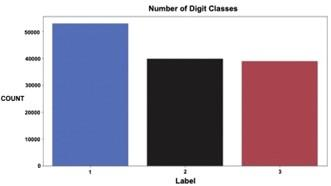Figure 7. EEG Pre-processed data label encoding

4.2 Sequence learning

EEG signals are time series signals of the brain's states and activities. This is the reason that sequential learning can be implemented on EEG data using various deep learning algorithms.

There are two types of Sequential Learning namely short-term and long-term. There are certain sets of problems that can effectively solve by recurrent neural networks ("RNN") utilizing the property of short-term memory, but it is unable to process very long sequences if using tanh or ReLU as an activation function. It also suffers from the Vanishing Gradient Problem .

Long Short-Term Memory (LSTM) is a kind of RNN that solves RNN problems. It handled the problem of long-term dependencies of RNN. LSTM can retain the information for a longer period. As shown in Figure 8, Information is retained by the cells and the memory manipulations are done by the gates.

## 8.jpg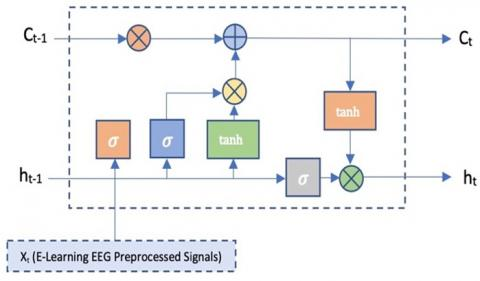Figure 8. Architecture of Long Short-Term Memory (LSTM)

LSTM architecture consists of Forget Gate, Input Gate, and Output Gate. Those gates are responsible for either ignoring or storing the previous output and generating output. In LSTM, with the help of sigmoid (σ), sum (+), multiplication (×), and hyperbolic tangent weights can easily be updated.

For EEG time series data, sequential learning may be defined using below Eqs. (1)-(6) as follows:

$n_t=\tanh \left(W_{\mathrm{n}}\left[\mathrm{h}_{\mathrm{t}-1}, x_{\mathrm{t}}\right]+b_{\mathrm{n}}\right)$               (1)

$i_t=\sigma\left(W_{\mathrm{i}}\left[\mathrm{h}_{\mathrm{t}-1}, \chi_{\mathrm{t}}\right]+b_{\mathrm{i}}\right)$               (2)

$f_t=\sigma\left(W_{\mathrm{f}}\left[\mathrm{h}_{\mathrm{t}-1}, \chi_{\mathrm{t}}\right]+b_{\mathrm{f}}\right)$               (3)

$C_t=C_{\mathrm{t}-1} \mathrm{f}_{\mathrm{t}}+n_{\mathrm{t}} \mathrm{i}_{\mathrm{t}}$               (4)

$U_t=\tanh \left(W_{\mathrm{u}} C_{\mathrm{t}-1} \,\,\,\,\, f_{\mathrm{t}}+b_{\mathrm{u}}\right)$               (5)

$V_t=\sigma\left(W_{\mathrm{v}}\left[\mathrm{h}_{\mathrm{t}-1}, x_{\mathrm{t}}\right]+b_{\mathrm{v}}\right)$               (6)

Let there be N features $\left\{x_1, x_2 \ldots x_N\right\}$, then $x_t$ is the input signal feature. Here long-term memory value=$C_{\mathrm{t}-1}$, short-term memory value=$h_{t-1}$, bias=$b_n$, weight matrix=$W_n$, ignore factor=$i_t$, forget factor $f_t$, $\mathrm{C}_{t-1} \mathrm{~F}_t$ is the output of forget gate, $n_t i_t$ is the output of learn gate, $C_t$ is the output of remember gate and $U_t V_t$ is the output of use gate .

4.3 Representation learning

In our work, we have used the 2DCNN model for E-learning EEG data classification as shown in Figure 9. Out of a total number of 131604 EEG samples, 118443 samples were used for model training purposes and 13161 samples were used for model testing purposes.

The mathematical convolution process is mentioned in below Eq. (7):

$a(t)=\left(x^* w\right)(\mathrm{t})=\int_{-\infty}^{\infty} x(b) \mathrm{w}(\mathrm{t}-\mathrm{b}) \mathrm{db}$               (7)

Our model is implemented with Python taking Keras deep learning APIs. The following table describes the hyperparameters used for the 2DCNN model as follows:

Table 1. Hyperparameters used in Convolution Neural Network (CNN)

 Hyperparameters Values Optimizer ADAM Loss Function Categorical Cross Entropy Metrics Accuracy Batch Size 250 Epochs 100 Dropout 0.25

As shown in Table 1, two Convolution layers with filters of sizes 8 and 16 were used along with rectified linear unit (ReLU) activation function, i.e., f(x)=max (0, x) respectively. Apart from this, Max Pooling is performed to reduce the dimensions for the next successive layer.

Finally, for the EEG classification, the SoftMax activation function is used. The dropout technique is also used to handle the problem of overfitting. Also, ADAM optimizer which is a stochastic gradient descent method used to handle sparse gradients .

## 9.jpg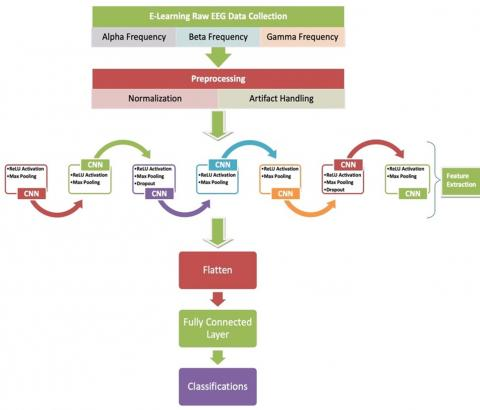Figure 9. Architecture of convolution neural network (CNN)

5. Result

The following graph Figure 10 refers to loss and accuracy curves for training and validation which represents Test Loss with respect to a number of epochs.

Our next Figure 11 represents the model loss during training. It can be seen that the accuracy of the model increases on each iteration of algorithms and model loss is decreasing at the same time with each iteration.

Below Figure 12 represents the confusion matrix, which is the best way to summarize the performance of our classification algorithm.

The two labels, i.e., Predicted labels and True labels clearly showed the errors made by the classifier. After the training of the 2DCNN model, the model was tested with the testing dataset. Then, the model is evaluated with the statistical performance measure parameters, i.e., True positive (TP), False positive (FP), False Negative (FN), and F-Measure .

Following represents the formulas used for the different performance measure parameters; Accuracy measured by ((TP+TN)/(FP+FN)), Precision measured by (TP/(TP+FP)), Recall measured by (TP/(TP+FN)), and F-Measure measured by ((2*Recall*Precision)/(Recall+Precision)). Table 2 represents the values of these performance measurement parameters for our 2DCNN model.

As mentioned in Table 2, after the end of model training and testing, the model got an accuracy of 70.34%, precision of 68.2%, Recall value of 69.8%, and F-measure value of 0.689.

## 10.jpg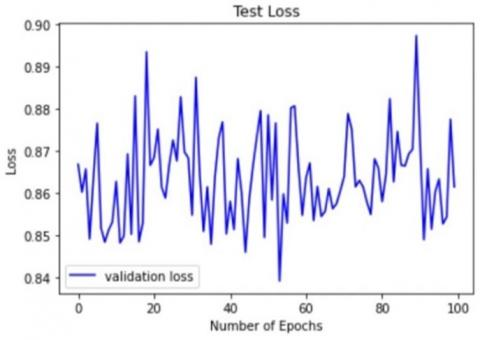Figure 10. Epochs Vs loss curve

## 11.pngFigure 11. Model loss during training

## 12.jpg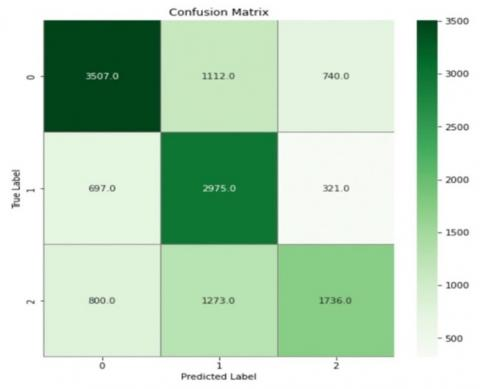Figure 12. Confusion matrix of trained CNN model

Our E-learning EEG data classification using 2DCNN has shown good results, but it may be better with the 2DLSTM approach. Because the EEG signals are kind of time series-based data and LSTM deep learning models have shown great results on time-series-based data. That is the primary reason for implementing the 2DLSTM network on the pre-processed 131604 EEG samples.

Again 118443 samples were used for model training purposes and 13161 samples were used for model testing purposes. Our model is implemented with Python taking Keras deep learning APIs. Following Table 3 describes the hyperparameters used for the 2DLSTM model as follows:

As shown in Table 3, two layers of LSTM each having 50 hidden cell units are used whose activations get sent forward to the next time step. For the EEG classification, the SoftMax activation function is used. Also, ADAM optimizer which is a stochastic gradient descent method used to handle sparse gradients.

Table 2. Performance parameters of trained model using CNN

 Performance Parameters Values Accuracy 70% Precision 68% Recall 70% F-Measure 0.68

Table 3. Hyperparameters used in Long Short-Term Memory (LSTM)

 Hyperparameters Values Optimizer ADAM Loss Function Categorical Cross Entropy Metrics Accuracy Batch Size 28 Epochs 100

Figure 13 refers to loss and accuracy curves for training and validation which represents Test Loss with respect to a number of epochs. As shown in Figure 12, test loss is exponentially decreasing as the model epochs increase.

## 13.jpg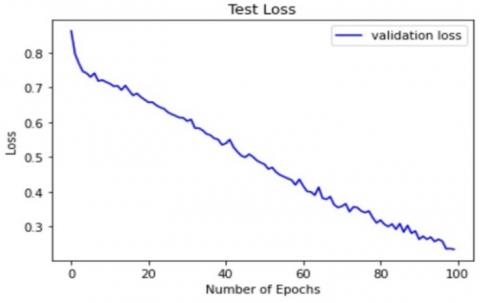Figure 13. Epochs Vs loss curve

## 14.png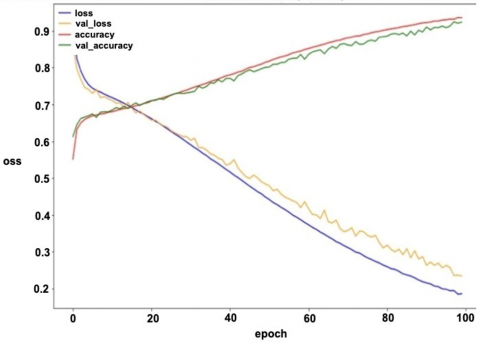Figure 14. Model loss during training

## 15.jpg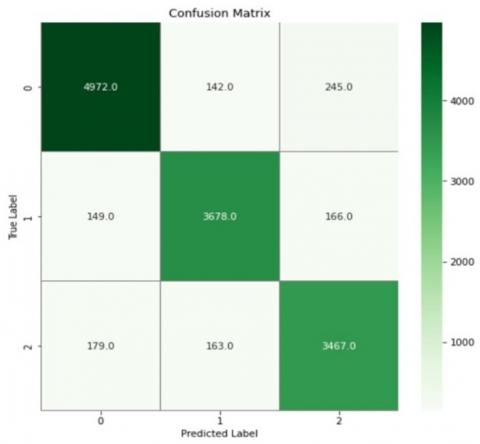Figure 15. Confusion matrix of trained LSTM model

Figure 14 represents the model loss during training. The accuracy of the model is increasing exponentially on each iteration of algorithms and model loss is decreasing at the same time with each iteration.

Figure 15 represents the confusion matrix for the 2DLSTM model, which is the best way to summarize the performance of our classification algorithm.

The two labels, i.e., Predicted labels and True labels clearly showed the errors made by the classifier which is quite less.

After the training of the 2DLSTM model, the model was tested with the testing dataset. Then, the model is evaluated with the statistical performance measure parameters, i.e., True positive (TP), False positive (FP), False Negative (FN), and F-Measure.

Following represents the formulas used for the different performance measure parameters; Accuracy measured by ((TP+TN)/(FP+FN)), Precision measured by (TP/(TP+FP)), Recall measured by (TP/(TP+FN)), and F-Measure measured by ((2*Recall*Precision)/(Recall+Precision)). Below Table 4 represents the values of these performance measurement parameters for our 2DLSTM model:

Table 4. Performance parameters of trained model using LSTM

 Performance Parameters Values Accuracy 93% Precision 92% Recall 89% F-Measure 0.90

As mentioned in above Table 4, after the end of model training and testing, the model got an accuracy of 93.81%, precision of 92.34%, Recall value of 89.40%, and F-measure value of 0.908.

6. Discussion

In our study, E-learning EEG data classification using the CNN and LSTM deep learning approaches was proposed. Comparative studies of both approaches based on the performance measure parameters are shown in below Table 5:

Table 5. Comparative analysis of CNN and LSTM models

 Performance Parameters CNN Trained Model Values LSTM Trained Model Values Accuracy 70% 93% Precision 68% 92% Recall 70% 89% F-Measure 0.68 0.90

It can be seen from the above Table 5 that the value of performance measure parameters, i.e., accuracy, precision, recall, and F-measure is higher in the case of the 2DLSTM approach as compared with the 2DCNN approach. A possible explanation for this is the EEG datasets represent the learning of participants while attending online classes and it is mostly time-series-based datasets. Since learning is continues and dependent on previously learned information.

The 2D LSTM model performs better than the 2D CNN model for EEG data classification because the LSTM model is better suited for capturing the temporal dynamics of EEG signals.

EEG signals are time series data, meaning that the signal at each time point is dependent on the previous time points. The 2D CNN model is designed to learn spatial features from 2D images, but it does not explicitly model the temporal dependencies between the input data. On the other hand, the 2D LSTM model is designed to model sequential data by maintaining a memory state that can capture the temporal dynamics of the input sequence.

## 16.pngFigure 16. Comparative study of latest EEG deep learning-based papers

## 17.jpg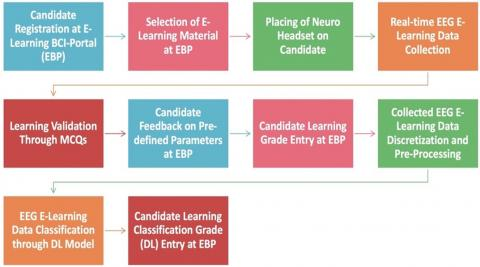Figure 17. Flow chart of EEG data collection & classification

The most important takeaway from our work is we have successfully classified the participant's learning based on real-time EEG data and it is far better than the conventional historical data processing techniques. Our study further proposes an automated framework that will provide customized recommendations to both learners as well as the E-learning forming authorities for their betterment. With our approach, participants' learning curve can be tracked which further help in finding the learning disabilities in the candidates.

Although there is no specific research has been conducted combining EEG with E-learning, some recent studies have been mentioned in the below Figure 16 targeting areas, i.e., Focus & Attention, Emotion Detection, Workload Detection, Mental Stages, etc.

Studies targeting the classification of Focus & Attention were able to achieve an accuracy of only 77% by implementing machine learning techniques with a limited dataset.

It can easily be seen the implementation of a deep learning algorithm in the EEG domain drastically boost the performance of classification, i.e., emotion detection using LSTM achieved 88% accuracy due to mainly its capability of automatic feature selection.

Figure 17 demonstrates the workflow of the recommended automated framework.

The following describes the important steps performed in the mentioned framework:

(1). Candidate Registration at E-learning BCI-Portal (EBP): Candidates will be registered with their basic education details to the portal.

(2). Selection of E-learning Material at EBP: It denotes the selection of a specific topic for the purpose of learning.

(3). Placing of Neuro Headset on Candidate: A neuro band will be placed on the participant's head for the purpose of EEG data collection.

(4). Real-time EEG E-learning Data Collection: Real-time EEG data will be stored while participants attend the online session.

(5). Learning Validation Through MCQs: Participants' learning will be validated through MCQs related to the attended topic and respective grades will also be stored.

(6). Candidate Feedback on Pre-defined Parameters at EBP: After the MCQs, participants' feedback regarding that session on a scale of 1 to 10 will be recorded which will further help with customized recommendations.

(7). Candidate Learning Grade Entry at EBP: Participant's learning grade i.e., Excellent, Good, Poor will be stored.

(8). Collected EEG E-learning Data Discretization and Pre-Processing: Then recorded EEG signals will be discretized through FFT and pre-processed for the trained DL models.

(9). EEG E-learning Data Classification through DL Model: After that pre-processed EEG data will be fed to DL models for classification purposes.

(10). Candidate Learning Classification Grade (DL) Entry at EBP: After the classification, the calculated Grade will be stored in a framework for further knowledge tracking and recommendations.

With the help of the above-mentioned framework, participants' learning curves along with sessions feedbacks and customized recommendations can be incorporated.

We have developed the E-learning BCI-Portal (EBP) as a solution of five major current E-learning problems that we have identified shown in Figure 18. It clearly shows the mapping of E-learning contents with their results along with the details of teaching methodology used in particular E-learning sessions.

The limitation of our study is that we have only focused on engineering students and with specific domains, i.e., computer science and application. In the future, we will try to accommodate other streams and courses also. Furthermore, the total EEG datasets collected is of 300+hours which is sufficient, but the deep learning algorithm works better with a large amount of data. So, further research on this domain may be recommended with diverse streams with large amounts of captured E-learning EEG-data. Future work related to E-learning EEG data may be extended for the identification of learning disabilities in the studied subjects and further recommending suitable treatments [27, 28].

## 18.jpgFigure 18. E-learning BCI-Portal (EBP)

7. Conclusions

There are many Online education platforms available offering courses in almost every domain. Our research work proposes a framework that validates the learning of participants and also provides recommendations in current teaching-learning scenarios that would be useful in better E-study materials, better tracking of candidates learning, and customized user-based recommendations for both users as well as content providers. This paper proposes deep learning models for classifying recorded real-time EEG-based E-learning data. The 2DLSTM model demonstrates better results compared to the 2DCNN model due to the time series nature of E-learning EEG data. In summary, the successful classification of participants' learning based on real-time EEG data has several practical advantages in education, psychology, and neurorehabilitation, including personalized learning, objective assessment of learning, early detection of learning difficulties, rehabilitation, and research. Future research work may also include investigating reasons, plausible treatments, and validation of the effects of treatment.

References

 Keskin, S. (2019). Factors affecting students’ preferences for online and blended learning: Motivational vs. cognitive. European Journal of Open, Distance and E-learning (EURODL), 22(2): 72-86.

 Shahzad, A., Hassan, R., Aremu, A.Y., Hussain, A., Lodhi, R.N. (2021). Effects of COVID-19 in E-learning on higher education institution students: The group comparison between male and female. Quality & Quantity, 55: 805-826. https://doi.org/10.1007/s11135-020-01028-z

 Zhao, F., Wu, Z., Li, G. (2023). Deep learning in cortical surface-based neuroimage analysis: A systematic review. Intelligent Medicine, 3(1): 46-58. https://doi.org/10.1016/j.imed.2022.06.002

 Valverde-Berrocoso, J., Garrido-Arroyo, M.D.C., Burgos-Videla, C., Morales-Cevallos, M.B. (2020). Trends in educational research about E-learning: A systematic literature review (2009-2018). Sustainability, 12(12): 5153. https://doi.org/10.3390/su12125153

 Pathak, D., Kashyap, R., Rahamatkar, S. (2022). A study of deep learning approach for the classification of electroencephalogram (EEG) brain signals. In Artificial Intelligence and Machine Learning for EDGE Computing. Academic Press, pp. 133-144. https://doi.org/10.1016/B978-0-12-824054-0.00009-5

 Obpaet, J., Paoprasert, N. (2020). Factors affecting engineering program performance using structural equation modeling technique. In 2020 International Symposium on Educational Technology (ISET). IEEE, pp. 201-205. https://doi.org/10.1109/ISET49818.2020.00051

 Arnicane, A., Souza, A.S. (2022). Tracking attentional states: Assessing the relationship between sustained and selective focused attention in visual working memory. Attention, Perception, & Psychophysics, 84(3): 715-738. https://doi.org/10.3758/s13414-021-02394-y

 Peng, C.J., Chen, Y.C., Chen, C.C., Chen, S.J., Cagneau, B., Chassagne, L. (2020). An EEG-based attentiveness recognition system using Hilbert-Huang transform and support vector machine. Journal of Medical and Biological Engineering, 40(2): 230-238. https://doi.org/10.1007/s40846-019-00500-y

 Yoshida, K., Takeda, K., Kasai, T., Makinae, S., Murakami, Y., Hasegawa, A., Sakai, S. (2020). Focused attention meditation training modifies neural activity and attention: Longitudinal EEG data in non-meditators. Social Cognitive and Affective Neuroscience, 15(2): 215-224. https://doi.org/10.1093/scan/nsaa020

 Salloum, S.A., Alhamad, A.Q.M., Al-Emran, M., Monem, A.A., Shaalan, K. (2019). Exploring students’ acceptance of E-learning through the development of a comprehensive technology acceptance model. IEEE Access, 7: 128445-128462. https://doi.org/10.1109/ACCESS.2019.2939467

 Mousavi, A., Mohammadi, A., Mojtahedzadeh, R., Shirazi, M., Rashidi, H. (2020). E-learning educational atmosphere measure (EEAM): A new instrument for assessing e-students' perception of educational environment. Research in Learning Technology, 28. https://doi.org/10.25304/rlt.v28.2308

 Singh, N., Gunjan, V.K., Zurada, J.M. (2022). Building SeisTutor intelligent tutoring system for experimental learning domain. In Cognitive Tutor: Custom-Tailored Pedagogical Approach. Singapore: Springer Nature Singapore, pp. 61-78. https://doi.org/10.1007/978-981-19-5197-8_4

 Onah, D.F., Pang, E.L., Sinclair, J.E., Uhomoibhi, J. (2021). An innovative MOOC platform: The implications of self-directed learning abilities to improve motivation in learning and to support self-regulation. The International Journal of Information and Learning Technology, 38(3): 283-298. https://doi.org/10.1108/IJILT-03-2020-0040

 Takeuchi, N. (2022). Perspectives on rehabilitation using non-invasive brain stimulation based on second-person neuroscience of teaching-learning interactions. Frontiers in Psychology, 12: 789637. https://doi.org/10.3389/fpsyg.2021.789637

 Lu, D.N., Le, H.Q., Vu, T.H. (2020). The factors affecting acceptance of E-learning: A machine learning algorithm approach. Education Sciences, 10(10): 270. https://doi.org/10.3390/educsci10100270

 Peterson, V., Galván, C., Hernández, H., Spies, R. (2020). A feasibility study of a complete low-cost consumer-grade brain-computer interface system. Heliyon, 6(3): e03425. https://doi.org/10.1016/j.heliyon.2020.e03425

 Pathak, D., Kashyap, R. (2022). Electroencephalogram-based deep learning framework for the proposed solution of E-learning challenges and limitations. International Journal of Intelligent Information and Database Systems, 15(3): 295-310. https://doi.org/10.1504/IJIIDS.2022.124081

 Xie, Z., Yu, X., Gao, X., Li, K., Shen, S. (2022). Recent advances in conventional and deep learning-based depth completion: A survey. IEEE Transactions on Neural Networks and Learning Systems, pp. 1-21. https://doi.org/10.1109/TNNLS.2022.3201534

 Li, J. (2021). Recent developments of deep learning in analyzing, decoding, and understanding neuroimaging signals. Frontiers in Neuroscience, 15: 652073. https://doi.org/10.3389/fnins.2021.652073

 Ko, W., Jeon, E., Jeong, S., Phyo, J., Suk, H.I. (2021). A survey on deep learning-based short/zero-calibration approaches for EEG-based brain-computer interfaces. Frontiers in Human Neuroscience, 15: 643386. https://doi.org/10.3389/fnhum.2021.643386

 Ghimire, A., Sekeroglu, K. (2022). Classification of EEG motor imagery tasks utilizing 2D temporal patterns with deep learning. In Proceedings of the 2nd International Conference on Image Processing and Vision Engineering (IMPROVE 2022), p. 182-188. https://doi.org/10.5220/0011069400003209

 Das, N., Zegers, J., Francart, T., Bertrand, A. (2020). Linear versus deep learning methods for noisy speech separation for EEG-informed attention decoding. Journal of Neural Engineering, 17(4): 046039. https://doi.org/10.1088/1741-2552/aba6f8

 Ni, J., Young, T., Pandelea, V., Xue, F., Cambria, E. (2023). Recent advances in deep learning based dialogue systems: A systematic survey. Artificial Intelligence Review, 56(4): 3055-3155. https://doi.org/10.1007/s10462-022-10248-8

 Zhang, X., Yao, L., Wang, X., Monaghan, J., Mcalpine, D., Zhang, Y. (2021). A survey on deep learning-based non-invasive brain signals: Recent advances and new frontiers. Journal of Neural Engineering, 18(3): 031002. https://doi.org/10.1088/1741-2552/abc902

 Rashid, M., Sulaiman, N., PP Abdul Majeed, A., Musa, R.M., Ab Nasir, A.F., Bari, B.S., Khatun, S. (2020). Current status, challenges, and possible solutions of EEG-based brain-computer interface: A comprehensive review. Frontiers in Neurorobotics, 25. https://doi.org/10.3389/fnbot.2020.00025

 Mansoor, A., Usman, M.W., Jamil, N., Naeem, M.A. (2020). Deep learning algorithm for brain-computer interface. Scientific Programming, 2020: 1-12. https://doi.org/10.1155/2020/5762149

 Edwards, B.I. (2021). Emerging trends in education: Envisioning future learning spaces and classroom interaction. Emerging Technologies for Next Generation Learning Spaces, 7-18. https://doi.org/10.1007/978-981-16-3521-2_2

 Chaki, J. (2022). Brain MRI segmentation using Deep Learning: Background Study and challenges. Brain Tumor MRI Image Segmentation Using Deep Learning Techniques, 1-12. https://doi.org/10.1016/b978-0-323-91171-9.00012-0